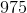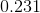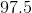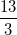## Example Questions

← Previous 1

### Example Question #16 : Proportion / Ratio / Rate

Sam can paint a house in three days while Dan can finish painting one in two days.  How long would it take to paint two houses if they worked together?

1.2 days

0.8 days

None of the answers are correct

2.4 days

1.0 day

2.4 days

Explanation:

In general for work problems:  W1 + W2 = 1 where Work = Rate x Time

Note, 1 represents the completed job assignment.

For example, W1 is the rate that the first person would finish the job multiplied by the time it would take two or more people to finish the job completely.

1/3x + 1/2x = 1 where x is the time it would take for both people to complete the job.

Find a common denominator to add the fractions, then solve for x.

x = 1.2 days for one house, but the questions asks about two houses, so the correct answer is 2.4 days.

### Example Question #17 : Proportion / Ratio / Rate

A farmer has a piece of property that is 10,000 feet by 40,000 feet.  His annual property taxes are paid at a rate of $3.50 per acre. If one acre = 43,560 ft2, how much will the farmer pay in taxes this year? Round to the nearest dollar. Possible Answers:$35,000

$31,500$3,214

$32,140$3,500

$32,140 Explanation: Property area = 10,000 ft x 40,000 ft = 400,000,000 ft2 Acreage = 400,000,000 ft2 / 43,560 ft2 per acre = 9,183 acres Taxes =$3.50 per acre x 9,183 acres = $32,140 ### Example Question #18 : Proportion / Ratio / Rate Hannah can travel to her destination in one of two ways: she can drive due north for 36 miles, then due west for 44 miles, traveling an average of 65 miles per hour. Or she can drive directly to the destination, heading northwest, traveling an average of 40 miles per hour. What is the difference, to the nearest minute, between the two routes? Possible Answers: 24 minutes 11 minutes 16 minutes 20 minutes 12 minutes Correct answer: 11 minutes Explanation: Remember that distance = rate x time For the first route, we can set up an equation where the total distance (36 + 44) equals the rate (65 mph) multiplied by the time: 36 +44 = 65t 80 = 65t t = 80/65 = 1.23 hrs = 1 hr, 14 min To find the time taken for the second route, we first figure out the distance traveled by using the Pythagorean Theorem. We know that the "legs" of the right triangle are 44 and 36, where the hypotenuse is the straightline distance (northwest), directly to the destination: a2+b2=c2 442+362=c2 3232=c2 c=56.85 56.85=40t 56.85/40=t t=1.42 hrs=1 hr, 25 min 1 hr. 25 min. – 1 hr. 14 min. = 11 min. ### Example Question #21 : Proportion / Ratio / Rate A motorcycle on a full tank of gas travels 478 miles. If a full tank of gas is 12 gallons, and gas costs$4.25 per gallon, what is the approximate miles per gallon rating of the motorcycle, and how much will a full tank of gas cost?

39.8, $51.00 39.8,$48.75

112.5, $51.00 112.5,$48.75

39.8, $51.00 Explanation: To calculate miles per gallon of gas, we take the 478 miles the motorcycle travels and divide it by the amount of gallons in a full tank of gas, 12 gallons. 478/12 = 39.83, or 39.8 when rounded to the tenths place For the price, we take the 12 gallons and multiply it by the amount that one gallon costs,$4.25.  (12)(4.25) = 51

### Example Question #21 : Proportion / Ratio / Rate

A car averages 31 miles per gallon. Currently, gas costs $3.69 per gallon. About how much would it cost in gas for this car to travel 3,149 miles? Possible Answers:$273.52

$101.58$374.83

$853.38 Correct answer:$374.83

Explanation:

First we determine how many gallons it will take to travel 3,149 miles with this particular car: 3,149/31=101.58 gallons. The cost of gas per gallon= $3.69, therefore 101.58x$3.69= $374.83. ### Example Question #23 : Proportion / Ratio / Rate A motorcycle averages 47 miles per gallon. If gas costs$4.13 per gallon, how much gas money is needed for a 1,457 mile road trip?

$570.50$356.06

$235.25$128.03

Not enough information given

$128.03 Explanation: We divide 1,457 miles by 47 miles per gallon to find that 31 gallons of gas are needed for the road trip. We then multiply the gallons of gas by the cost per gallon to find: 31 x 4.13 = 128.03 ### Example Question #24 : Proportion / Ratio / Rate If a car averages 32 miles per hour, how far will it go in 20 minutes (rounded to the nearest tenth of a mile)? Possible Answers: 32.0 miles 10.6 miles 12.3 miles 10.7 miles 10.0 miles Correct answer: 10.7 miles Explanation: First, we need to convert the 1 hour into minutes in order to keep consistent with units -- so, the car averages 32 miles per 60 minutes. Then, a ratio can be set up to solve this: 32 mi / 60 min = x mi / 20 min. Cross multiplying and dividing, we get x = 10.667 miles. Rounding to the nearest tenth, this becomes 10.7 miles. ### Example Question #25 : Proportion / Ratio / Rate A new car can travel an average of 63 miles per gallon of gasoline. Gasoline costs$5.05 per gallon. How much would it cost to travel 6,363 miles in this car?

$405.05$101

$510.05$505

$510 Correct answer:$510.05

Explanation:

First, find the total amount of gas necessary for the trip. 6363/63 = 101 gallons (easy to see as 63 * 100 = 6300 + 1 * 63 = 6363). Then multiply the number of gallons by the price per gallon of gasoline, 5.05 * 101 = \$510.05 and is your answer (again, easy to see when 5.05 * 100 + 1 * 5.05).

### Example Question #26 : Proportion / Ratio / Rate

If Denise drives at a constant rate of 65 mph for 15 hours, how far will she drive in miles?Explanation:

Remember that distance/time=rate, so then:

x/15 = 65

x = 65 * 15

x = 975 miles

### Example Question #27 : Proportion / Ratio / Rate

Joe and Jake canoed down stream in 30 minutes and then up stream in 60 minutes.  How fast were they paddling if the river current is 3 mph?

9 mph

3 mph

None of the answers are correct

5 mph

7 mph